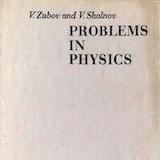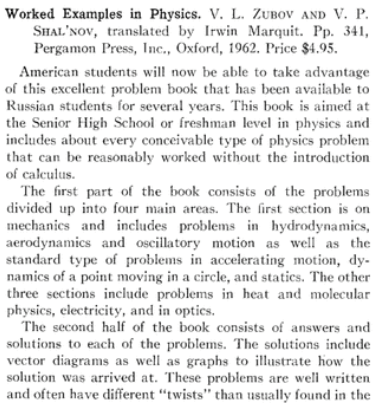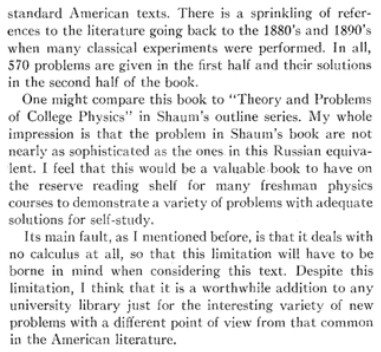# Problems in Physics by V. Zubov and V. ShalnovProblems in Physics by V. Zubov and V. Shalnov was published by MIR publishers (Russia) in 1974. The book has 570 problems that can be solved without calculus. The book is divided into two parts. The first part of the book consists of the problems from - mechanics, heat and molecular physics, electricity, and optics. The second part of the book consists of answers and solutions to these problems. The book includes a variety of problems with adequate solutions for self-study. The book is recommended for physics lovers. Its utility for IIT JEE is limited due to (1) not having problems involving calculus (2) having many problems beyond JEE syllabus. IIT-JEE aspirants shall keep an eye on JEE syllabus while solving problems from this book.

## Key Features

The book consists of problems discussed in tutorial sessions of physics olympiad at Moscow University. The problems in this book focus on those physical questions, situations, and laws where students are likely to make mistakes. The concepts and formulae are briefly described in each section. The number of problems in each section is

1. Mechanics - 221 problems
2. Heat and Molecular Physics - 72 problems
3. Electricity - 190 problems
4. Optics - 87 problems

Many problems are intended to explain the laws which students often fail to grasp properly. Some problems are beyond the school syllabus. The most difficult problems and the problems outside the syllabus are provided with detailed explanations in order to give the student a better understanding of the general principles of the solution. Some sections also have brief information about the most frequent mistakes and the simplest means of solution.

In all, 570 problems are given in the first half and their solutions in the second half of the book.